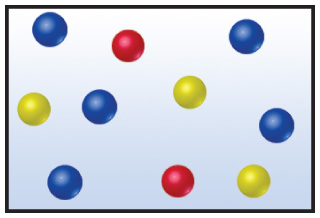# Solution: The drawing below represents a mixture of three different gases.Rank the three components in order of decreasing partial pressure.

###### Problem

The drawing below represents a mixture of three different gases.Rank the three components in order of decreasing partial pressure.

###### Solution
• Partial pressure is relative to the number of moles or molecules gases.
• Raoult's law confirms this where:

Pgas = (total P of gases)(mole fraction of gas)

• It can be seen that P of gas directly proportional to the mole fraction of the gas, the higher the # of moles, the higher the partial pressureView Complete Written Solution